Courses

# Previous year questions (2014-20) - Thermodynamics Class 11 Notes | EduRev

## NEET : Previous year questions (2014-20) - Thermodynamics Class 11 Notes | EduRev

The document Previous year questions (2014-20) - Thermodynamics Class 11 Notes | EduRev is a part of the NEET Course Chemistry Class 11.
All you need of NEET at this link: NEET

Q.1. An increase in the concentration of the reactants of a reaction leads to change in :     
(a) threshold energy
(b) collision frequency
(c) activation energy
(d) heat of reaction
Ans:
b
Solution: Collision frequency, as number of molecules per unit volume increases.

(a) q < 0, ΔT = 0 and w = 0
(b) q > 0, ΔT > 0 and w > 0
(c) q = 0, ΔT = 0 and w = 0
(d) q = 0, ΔT < 0 and w > 0
Ans:
c
Solution: For an adiabatic process, q = 0
∴ w = 0
& from First law of Thermodynamics, ΔE = 0
or ΔT = 0

Q.3. For the reaction, 2Cl(g) → Cl2(g), the correct option is:      
(a) ΔrH < 0 and ΔrS > 0
(b) ΔrH < 0 and ΔrS < 0
(c) ΔrH > 0 and ΔrS > 0
(d) ΔrH > 0 and ΔrS < 0
Ans:
b
Solution:
2Cl(g) → Cl2(g) + Heat
Due to bond formation stability increases which results in release of heat.
∴ ΔHr = -ve or exothermic process
ΔHr < O
& ΔS < O, because number of Cl atoms decreases in the formation of Cl2(g)

Q.4. In which of the following processes, heat is neither absorbed nor released by a system?    
(a) Isothermal
(c) Isobaric
(d) Isochoric
Ans:
b
Solution:
In adiabatic process, there is no exchange of heat.

Q.5. A sample of 0.1 g of water at 100°C and normal pressure (1.013 x 105 Nm–2) requires 54 cal of heat energy to convert to steam at 100°C. If the volume of the steam produced is 167.1 cc, the change in internal energy of the sample, is:-    
(a) 104.3 J
(b) 208.7 J
(c) 42.2 J
(d) 84.5 J
Ans:
c
Solution: ΔQ = 54 cal = 54 × 4.18 joule = 225.72 joule
ΔW = P[Vsteam – Vwater] [For water 0.1 gram=0.1 cc]
= 1.013 × 105[167.1 × 10–6 – 0.1 × 10–6] joule
= 1.013 × 167 × 10–1 = 16.917 joule
By FLOT
ΔU = ΔQ – ΔW = 225.72 – 16.917
ΔU = 208.8 joule

Q.6. The volume (V) of a monatomic gas varies with its temperature (T), as shown in the graph. The ratio of work done by the gas, to the heat absorbed by it, when it undergoes a change from state A to state B, is:-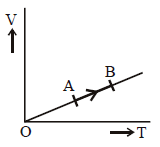(a) 2/5
(b) 2/3
(c) 1/3
(d) 2/7
Ans:
a
Solution: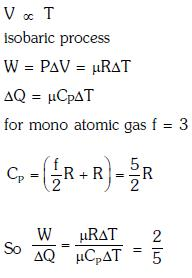Q.7. The efficiency of an ideal heat engine working between the freezing point and boiling point of water, is:-    
(a) 26.8%
(b) 20%
(c) 6.25%
(d) 12.5%
Ans:
a
Solution: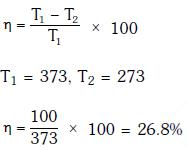Q.8. Thermodynamic processes are indicated in the following diagram: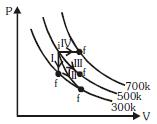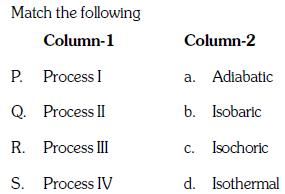(a) P → c, Q → a, R → d, S → b
(b) P → c, Q → d, R → b, S → a
(c) P → d, Q → b, R → a, S → c
(d) P → a, Q → c, R → d, S → b
Ans:
a
Solution:
Process (1) → volume constant → Isochroic
Process (3) → Temperature constant → Isothermal
Process (4) → Pressure constant → Isobaric

Q.9. A carnot engine having an efficiency of 1/2 as heat engine, is used as a refrigerator. If the work done on the system is 10 J, the amount of energy absorbed from the reservoir at lower temperature is:-    
(a) 90 J
(b) 99 J
(c) 100 J
(d) 1 J
Ans:
a
Solution: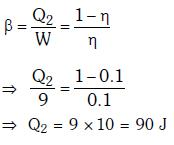Q.10. A refrigerator works between 40 celcius and 300 celcius. It is required to remove 600 calories of heat every second in order to keep the temperature of the refrigerated space constant. The power required is :(Take 1 cal = 4.2 Joules)    
(a) 2365W
(b) 2.365W
(c) 23.65W
(d) 236.5W
Ans:
d
Solution:
Given,
Temperature of the source, T = 30o C = 30 + 273  = 303 K
Temperature of sink, T2 = 4o C = 4 + 273 = 277 K
We know,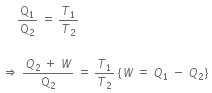where, Q2 is the amount of heat drawn from the sink at T2,
W is the work done on the working substance,
Q1 is the amount of heat rejected to source at room temperature T1.
That is,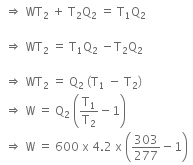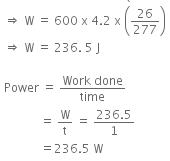Q.11. A gas is compressed isothermally to half its initial volume. The same gas is compressed separately through an adiabatic process until its volume is again reduced to half. Then    
(a) Which of the case (whether compression through isothermal or through adiabatic process) requires more work will depend upon the atomicity of the gas
(b) Compressing the gas isothermally will require more work to be done
(c) Compressing the gas through adiabatic process will require more work to be done
(d) Compressing the gas isothermally or adiabatically will require the same amount of work
Ans:
c
Solution: Directly from graph the magnitude of work done = Area under p-v plot is larger for adiabatic compression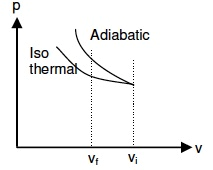Q.12. One mole of an ideal diatomic gas undergoes a transition from A to B along a path AB as shown in the figure,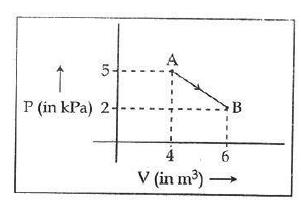The change in internal energy of the gas during the transition is :    
(a) -12 kJ
(b) 20 KJ
(c) - 20 KJ
(d) 20 J
Ans:
c
Solution: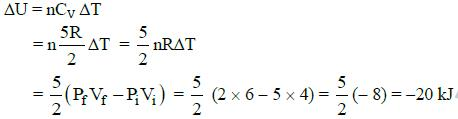Q.13. A Carnot engine, having an efficiency of η = 1/10 as heat engine, is used as a refrigerator. If the work done on the system is 10 J, the amount of energy absorbed from the reservoir at lower temperature is:    
(a) 1 J
(b) 100 J
(c) 99 J
(d) 90 J
Ans: d

Solution: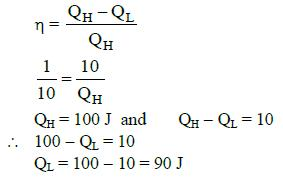Q.14. Figure below shows two paths that may be taken by a gas to go from a state A to a state C.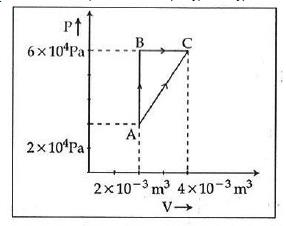In process AB, 400 J of heat is added to the system and in process BC, 100 J of heat is added to the system. The heat absorbed by the system in the process AC will be:    
(a) 300 J
(b) 380 J
(c) 500 J
(d) 460 J
Ans:
d
Solution: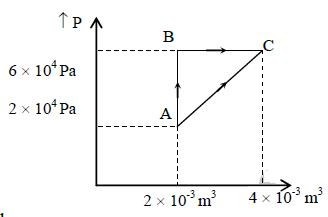See figure alongside
Process AB is isochoric so no work is done.
Heat added to be system is Q = 400 J.
Q = ΔU + ΔW
where ΔU is the change in internal energy
ΔW is the work done.
Since ΔW = 0
ΔU = Q = 400 J
Change in internal energy is 400 J.
Process BC is isobaric and the work done is given by
ΔW = P(V2 -V1) = 6 x 104(4 x 10-3 - 2 x 10-3)
= 6 x 104 x 2 x 10-3 = 120 J
Heat added to be system is Q = 100 J.
Since Q = ΔU + ΔW
∴ ΔU = Q- ΔW = (100 - 120) J = -20 J
Change in internal energy is - 20 J.Total increase in internal energy is going from state A to state C is 400 - 20 = 380 J
Work done in process AC is the area under the curve Area of the trapezium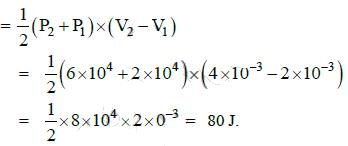Since Q = ΔU + ΔW
and ΔU the change in internal energy in process AC, we have
ΔU = 380 J and ΔW = 80 J
∴ Q = ΔU + ΔU = 380 + 80 = 460 J

Q.15. A monoatomic gas at a pressure P, having a volume V expands isothermally to a volume 2v and then adiabatically to a volume 16v. The final pressure of the gas is: (Take γ = 5/3)    
(a) P/64
(b) 16P
(c) 64P
(d) 32P
Ans:
a
Solution: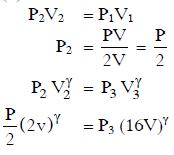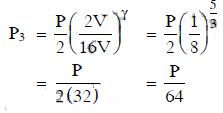Q.16. A thermodynamics system undergoes cyclic process ABCDA as shown in fig. The work done by the system in the cycle is: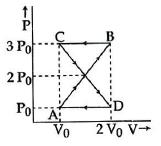(a) P0V0/2
(b) zero
(d)  2P0V0
Ans:
b
Solution: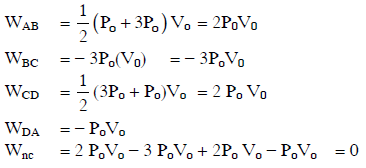Offer running on EduRev: Apply code STAYHOME200 to get INR 200 off on our premium plan EduRev Infinity!

## Chemistry Class 11

136 videos|245 docs|180 tests

,

,

,

,

,

,

,

,

,

,

,

,

,

,

,

,

,

,

,

,

,

;